# DM - Functions & Relations -Q14

+1 vote

Let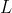be the set of all propositions generated by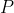and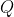(Whereandare Two Propositions) using at most one of the following logical operations :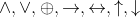, Where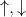are NAND and NOR operators respectively.

i.e.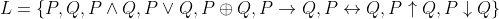A Relation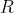is defined onsuch that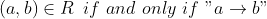is Tautology.

The relation R is

A. An equivalence relation

B. Not an equivalence relation because R is Not transitive.

C. A partial order relation

D. Not a partial order relation because R is Not anti-symmetric.asked Jun 24
reshown 6 days agoanswered 4 days ago by (112,390 points)